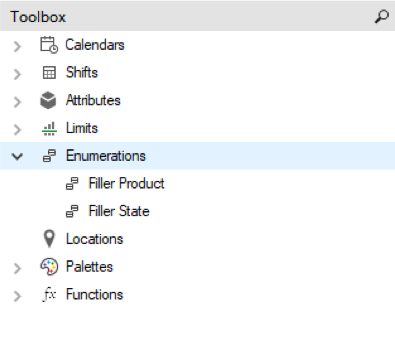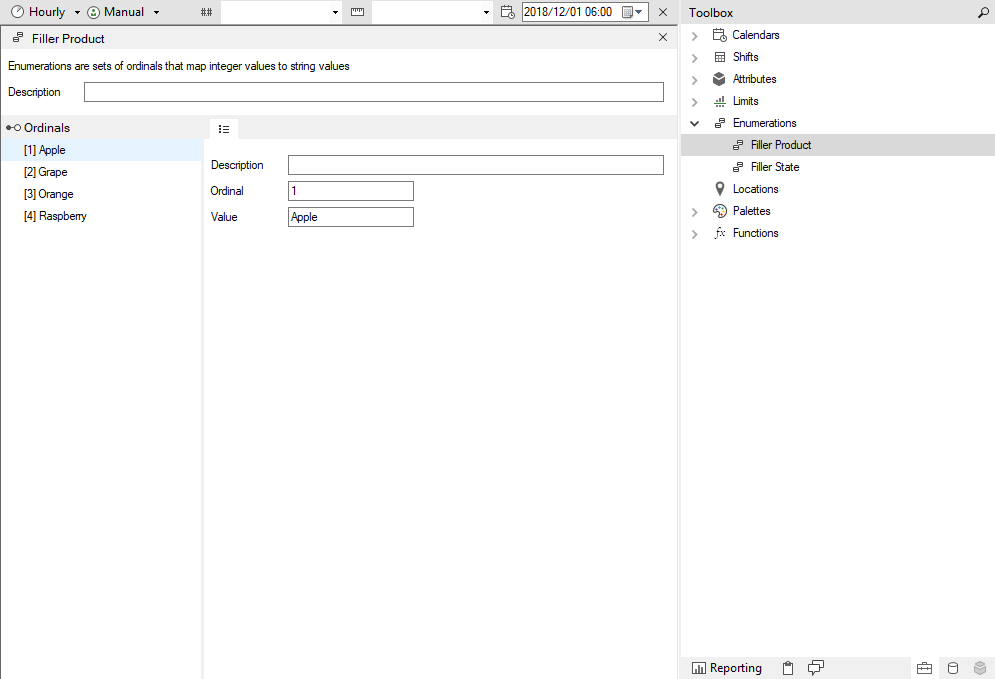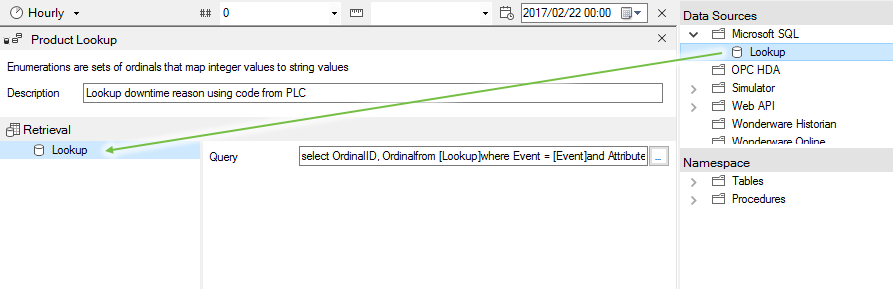Follow

# What Are "Enumerations", and what is the difference between a "Static Enumeration" and a "Lookup Enumeration"?

An Enumeration is a set of ordinals that map integer values to string values. Think of Enumerations as “Lookups”.For example, in your Historian, you may have an integer tag called “FL001.Product” that changes between 0 and 4.

0 represents an “Idle” state for the Filler, but 1 to 4 represent the various products or brands that your Juice Factory produces:

0 = No Production
1 = Apple
2 = Grape
3 = Orange
4 = Raspberry

There are two types of Enumerations that can be configured:

Note: Enumerations are used in conjunction with Event Attributes

### Static EnumerationsA static enumeration consists of a fixed list of ordinals defined with the following properties:

 Description This is the ordinal’s description. Ordinal This is the integer value that Flow associates with the ordinal’s string “Value”. Value This is the string value that is mapped by Flow to the ordinal.

### Lookup Enumeration

The Lookup Enumeration type allows you to query a Data Source for ordinal values. For example, you could query a Microsoft SQL database for the ordinal and value pairs. The Lookup Enumeration is dynamic and will change depending on the underlying data source values.When using the Microsoft SQL data source, your query must return two columns, namely “Ordinal” (integer) and “Value” (string). Your query can contain the following optional placeholders:

 [Event] The name of the event that is using this Lookup Enumeration. [Attribute] The name of the attribute that is using this Lookup Enumeration [Ordinal] The integer Ordinal returned by the Attribute value query. [Timestamp] The date and time for which the attribute is evaluated.

The following is an example SQL query for a “Lookup” Enumeration:

```select Ordinal, Value from Lookup
where Event = ‘[Event]’
and Attribute = ‘[Attribute]’
and Ordinal = [Ordinal]
```